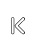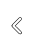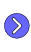## Exercises

#### 1. Fourier series

• Set up a figure 400 x 400 points. Centre the coordinate system, and choose a scale of 1 cm. Draw the axes. Then draw a square-wave.
• Graph the first approximation to the square wave 2 sin(2 pi x)/pi.
• Draw the approximation by 9 terms. The n-th term is (2/pi) sin (2 pi (2n-1) x)/(2n-1).
• Now make a series of pages, amounting to a simple animation.#### 2. Graphing integrals

• Let F(x) be the definite integral of cos t2 from 0 to x. (This is called the Fresnel integral.) Graph F(x) for x = 0 to 24. Use Simpson's rule and Bézier curves. (Look at what the manual says about Bézier curves and derivatives.)

#### 3. Newton's method

• Put together one or more figures that, briefly and all by themselves, explain Newton's method for finding roots.